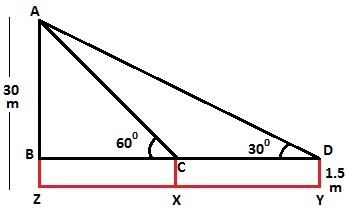Guru

# A 1.5 m tall boy is standing at some distance from a 30 m tall building. The angle of elevation from his eyes to the top of the building increases from 30° to 60° as he walks towards the building. Find the distance he walked towards the building. Q.6

• 0

Sir give me the best way to solve the problem form class 10th ncert of Some applications of Trigonometry chapter of exercise 9.1 of question no.6, this is an important question for board exams so give me the simple way to solve this problem A 1.5 m tall boy is standing at some distance from a 30 m tall building. The angle of elevation from his eyes to the top of the building increases from 30° to 60° as he walks towards the building. Find the distance he walked towards the building.

Share

1. Let the boy initially stand at point Y with inclination 30° and then he approaches the building to the point X with inclination 60°.To Find: The distance boy walked towards the building i.e. XY

From figure,

XY = CD.

Height of the building = AZ = 30 m.

AB = AZ – BZ = 30 – 1.5 = 28.5

Measure of AB is 28.5 m

In right ΔABD,

tan 30° = AB/BD

1/√3 = 28.5/BD

BD = 28.5√3 m

Again,

In right ΔABC,

tan 60° = AB/BC

√3 = 28.5/BC

BC = 28.5/√3 = 28.5√3/3

Therefore, the length of BC is 28.5√3/3 m.

XY = CD = BD – BC = (28.5√3-28.5√3/3) = 28.5√3(1-1/3) = 28.5√3 × 2/3 = 57/√3 = 19√3  m.

Thus, the distance boy walked towards the building is 19√3 m.

• 1